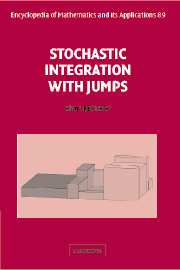Home Newsletter News BooksLanguage/LinguaBooks of Mathematics· Book News · Most clicked · Least clicked · Books Index · Search on Amazon

Search for a Book
Add a new BookStochastic Processes

 Probability and Statistics -> Stochastic Processes Search on Amazon

An Introduction To Stochastic Modeling
Author: Howard M.Taylor Samuel Karlin  Language:This book is intended as a beginning text in stochastic processes for stu- dents familiar with elementary probability calculus. Its aim is to bridge the gap between basic probability know-how and an . . . . .
Stochastic Integration And Stochastic Differential Equations
Author: Klaus Bichteler  Language:Contents: Introduction; Integrators and Martingales; Extension of the Integral; Control of Integral and Integrator; Stochastic Differential Equations; Complements to Measure Theory.
Probability Theory and Stochastic Processes with Applications
Author: Oliver Knill  Language:These notes grew from an introduction to probability theory taught during the first and second term of 1994 at Caltech. There was a mixed audience of undergraduates and graduate students in the firs . . . . .
Probability and Stochastic Processes with Applications
Author: Oliver Knill  Language:These notes grew from an introduction to probability theory taught during the first and second term of 1994 at Caltech. There was a mixed audience of undergraduates and graduate students in the firs . . . . .
Applied Stochastic Processes in science and engineering
Author: M. Scott  Language:This book is designed as an introduction to the ideas and methods used to formulate mathematical models of physical processes in terms of random functions. The rst ve chapters use the historical d . . . . .
Probability and Stochastic Processes with Applications
Author: Oliver Knill  Language:Contents: Introduction; Limit Theorems; Discrete stochastic processes; Continuous stochastic processes; Selected topics.
Introduction to Stochastic Processes
Author: G. F. Lawler  Language:Emphasizing fundamental mathematical ideas rather than proofs, Introduction to Stochastic Processes, Second Edition provides quick access to important foundations of probability theory applicable to p . . . . .
Lectures on Stochastic Processes
Author: K. Ito  Language:Contents:Preliminaries; Markov Processes; Srong Markov Processes; Multi-dimensional Brownian Motion; Additive Processes; Stochastic Differential Equations; Linear Diffusion.
Large deviations for stochastic processes
Author: By Jin Feng and Thomas G. Kurtz  Language:Contents: Introduction; Overview; Large deviations and exponential tightness; Large deviations for stochastic processes; Large deviations for Markov processes and nonlinear semigroup convergence; La . . . . .
An Introduction to Stochastic Differential Equations
Author: Lawrence Craig Evans  Language:Contens: Introduction; A crash course in basic probability theory; Brownian motion and white noise; Stochastic integrals, Itos formula; Stochastic differential equations.
Lectures on Stochastic Analysis
Author: Thomas G.Kurtz  Language:Contents: Review of probability; Continuous time stochastic processes; Martingales; Stochastic integrals; Covariation and Its formula; Stochastic Differential Equations; Stochastic differential . . . . .
Lectures on Stochastic Differential Equations and Malliavin Calculus
Author: S. Watanabe  Language:These notes are based on six-week lectures given at T.I.F.R. Centre, Indian Institute of Science, Bangalore, during February to April, 1983. My main purpose in these lectures was to study solutions o . . . . .
Introduction to Stochastic Processes
Author: Gordan itković  Language:Contents:Probability review; Mathematica in 15 minutes; Stochastic Processes; The simple random walk; Generating functions; Random walks - advanced methods; Branching processes; Markov Chains; The . . . . .
Introduction to the theory of stochastic processes and Brownian motion problems
Author: J. L. Garcia-Palacios  Language:These notes are an introduction to the theory of stochastic processes based on several sources. The presentation mainly follows the books of van Kampen and Wio, except for the introduction, which is t . . . . .
Stochastic Calculus and Stochastic Filtering
Author: Alan Bain  Language:These notes provide a fairly complete elementary introduction to the basics of stochastic integration with respect to continuous semimartingales (not just with respect to a Brownian Motion). They cont . . . . .
Stochastic Processes
Author: S.R.S.Varadhan  Language:Contents: Regularity of stochastic processes; Convergence of random walks to Brownian motion; Brownian motion and its martingales; Diﬀusion processes; Stochastic Integrals; Its formula; . . . . .

Add a new book
```Home |  Authors | About | Contact Us |  Email